Related Articles

# Get Minutes from timestamp in Pandas-Python

• Last Updated : 17 Aug, 2020

Pandas is an open-source library built for Python language. It offers various data structures and operations for manipulating numerical data and time series.

Here, let’s use some methods provided by pandas to extract the minute’s value from a timestamp.

Method 1: Use of pandas.Timestamp.minute attribute.

This attribute of pandas can be used to extract the minutes from a given timestamp object.

Example1:
Let’s first create a timestamp object below:

## Python3

 `# import pandas library``import` `pandas as pd ``   ` `# create a Timestamp object ``time_stamp ``=` `pd.Timestamp(``2020``, ``7``, ``20``,``                          ``12``, ``41``, ``32``, ``15``) ``   ` `# view the created time_stamp``print``(time_stamp)`

Output: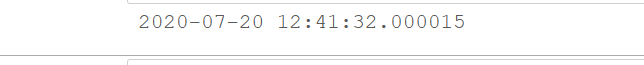In the above-created timestamp object, the minute’s value is “41”. Let’s extract this value using the Timestamp.minute attribute.

## Python3

 `# display the value of minute from``# the created timestamp object``print``(time_stamp.minute)`

Output:Example 2:

Create a timestamp object:

## Python3

 `# import pandas library``import` `pandas as pd ``   ` `# create a Timestamp object ``time_stamp ``=` `pd.Timestamp(``2020``, ``7``, ``20``) ``   ` `# view the created time_stamp``print``(time_stamp)`

Output: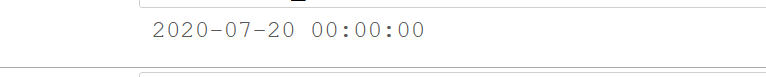In the above-created timestamp object, the minute’s value is “0”. Let’s extract this value using the Timestamp.minute attribute.

## Python3

 `# display the value of minute from``# the created timestamp object``print``(time_stamp.minute)`

Output: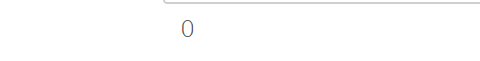Method 2: Use of Series.dt.minute attribute.

Now, consider the example of a pandas dataframe with one of the columns containing timestamps. In this case, we would first use the Series.dt method to access the values of the series as a DateTime object and then use the minute attribute to extract the minutes from the datetimes object.

Example 1:
First, create a pandas dataframe:

## Python3

 `# import pandas library``import` `pandas as pd `` ` `# create a series``sr ``=` `pd.Series([``'2020-7-20 12:41'``, ``                ``'2020-7-20 12:42'``, ``               ``'2020-7-20 12:43'``,``                ``'2020-7-20 12:44'``])`` ` `# convert the series to datetime``sr ``=` `pd.to_datetime(sr)`` ` `# create a pandas dataframe with a``# column having timestamps``df ``=` `pd.DataFrame(``dict``(time_stamps ``=` `sr))``   ` `# view the created dataframe``print``(df)`

Output: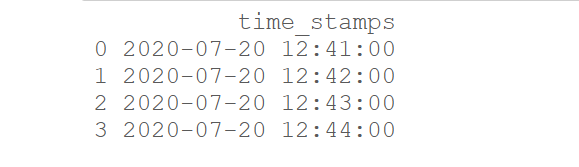Extracting the minute from each of the timestamp in the dataframe:

## Python3

 `# extract minutes from time stamps and``# add them as a separate column``df[``'minutes_from_timestamps'``] ``=` `df[``'time_stamps'``].dt.minute`` ` `# view the updated dataframe``print``(df)`

Output: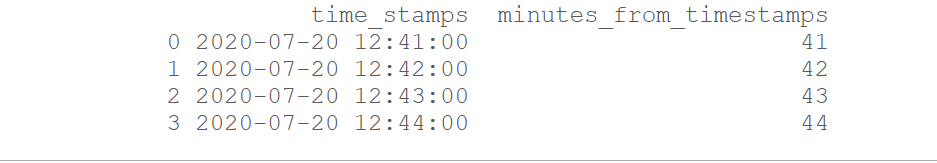Example 2:

Create a pandas dataframe:

## Python3

 `# import pandas library``import` `pandas as pd `` ` `# create a series``sr ``=` `pd.Series(pd.date_range(``'2020-7-20 12:41'``, ``                             ``periods ``=` `5``,``                             ``freq ``=` `'min'``))`` ` `# create a pandas dataframe with a``# column having timestamps``df ``=` `pd.DataFrame(``dict``(time_stamps``=``sr))``   ` `# view the created dataframe``print``(df)`

Output: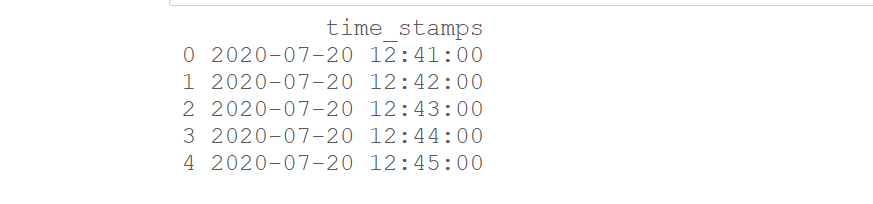Extracting the minute from each of the timestamp in the dataframe:

## Python3

 `# extract minutes from time stamps and``# add them as a separate column``df[``'minutes_from_timestamps'``] ``=` `df[``'time_stamps'``].dt.minute`` ` `# view the updated dataframe``print``(df)`

Output: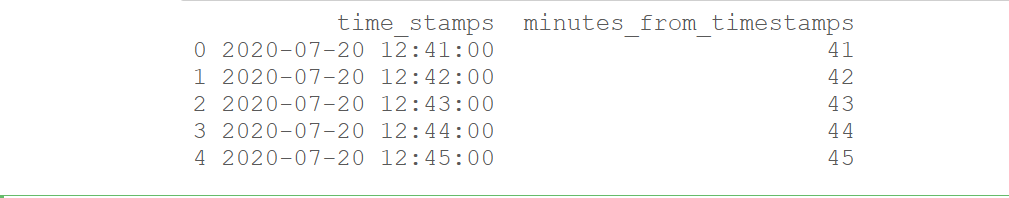Attention geek! Strengthen your foundations with the Python Programming Foundation Course and learn the basics.

To begin with, your interview preparations Enhance your Data Structures concepts with the Python DS Course. And to begin with your Machine Learning Journey, join the Machine Learning – Basic Level Course

My Personal Notes arrow_drop_up